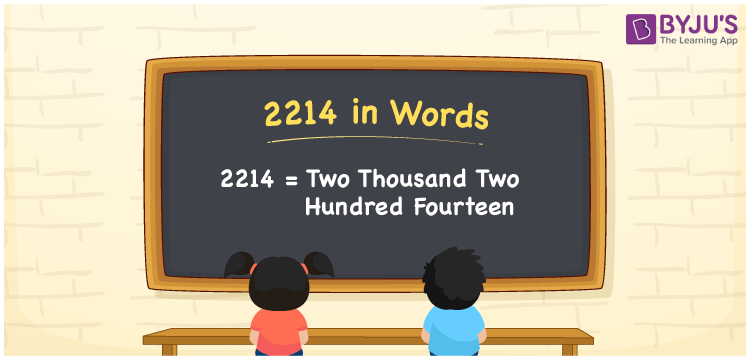# 2214 in words

2214 in words is written as Two Thousand Two Hundred and Fourteen. 2214 represents the count or value. The article on Counting Numbers can give you an idea about count or counting. The number 2214 is used in expressions that relate to money, distance, length, year and others. Let us consider an example for 2214. ”The electricity bill for the month of February was Two Thousand Two Hundred and Fourteen rupees.”

 2214 in words Two Thousand Two Hundred and Fourteen Two Thousand Two Hundred and Fourteen in Numbers 2214

## 2214 in English Words## How to Write 2214 in Words?

We can convert 2214 to words using a place value chart. The number 2214 has 4 digits, so let’s make a chart that shows the place value up to 4 digits.

 Thousands Hundreds Tens Ones 2 2 1 4

Thus, we can write the expanded form as:

2 × Thousand + 2 × Hundred + 1 × Ten + 4 × One

= 2 × 1000 + 2 × 100 + 1 × 10 + 4 × 1

= 2214

= Two Thousand Two Hundred and Fourteen.

2214 is the natural number that is succeeded by 2213 and preceded by 2215.

2214 in words – Two Thousand Two Hundred and Fourteen.

Is 2214 an odd number? – No.

Is 2214 an even number? – Yes.

Is 2214 a perfect square number? – No.

Is 2214 a perfect cube number? – No.

Is 2214 a prime number? – No.

Is 2214 a composite number? – Yes.

## Solved Example

1. Write the number 2214 in expanded form

Solution: 2 × 1000 + 2 × 100 + 1 × 10 + 4 × 1

We can write 2214 = 2000 + 200 + 10 + 4

= 2 × 1000 + 2 × 100 + 1 × 10 + 4 × 1

## Frequently Asked Questions on 2214 in words

Q1

### How to write 2214 in words?

2214 in words is written as Two Thousand Two Hundred and Fourteen.
Q2

### Is 2214 a perfect square number?

No. 2214 is not a perfect square number.
Q3

### Is 2214 a prime number?

No. 2214 is not a prime number.